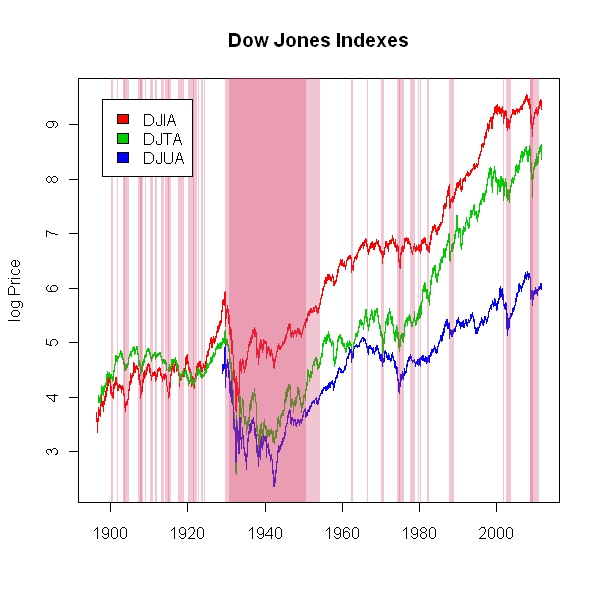Want to share your content on R-bloggers? click here if you have a blog, or here if you don't.

Drawdown is my favorite measure of risk.  It picks up extended autocorrelated pain often not seen in risk measures, and best illustrates frustration, panic, and loss of confidence (Drawdown Control Can Also Determine Ending Wealth).  I thought I should try some new ways to see it in R.  This first graph uses the zoo package to show 20% drawdowns in light pink and 40% drawdowns in darker pink.From TimelyPortfolio

PerformanceAnalytics makes drawdown graphs and overlays very easy, but for some reason I got an error in findDrawdowns, so I instead used the table.Drawdowns function to see the 5 worst drawdowns.From TimelyPortfolioFrom TimelyPortfolio

`require(quantmod)require(PerformanceAnalytics)   getSymbols("DJIA",src="FRED")getSymbols("DJTA",src="FRED")getSymbols("DJUA",src="FRED")   DJ <- merge(DJIA,DJTA,DJUA)   #DJ.yearly <- DJ[endpoints(DJ, on="years", k=1),]DJ.roc <- ROC(DJ,n=1,type="discrete")DJ.draw = Drawdowns(DJ.roc)   #jpeg(filename="DJ plot with Drawdowns zoo.jpg",#	quality=100,width=6.25, height = 6.25,  units="in",res=96)plot.zoo(log(DJ),plot.type="single",	col=c(2,3,4),ylab="log Price",xlab=NA,main="Dow Jones Indexes")rgb <- hcl(c(0, 0, 260), c = c(100, 0, 100), l = c(50, 90, 50), alpha = 0.3)xblocks(index(DJ),as.vector(DJ.draw[,1] < -0.20),col = rgb)xblocks(index(DJ),as.vector(DJ.draw[,1] < -0.40),col = rgb)legend("topleft",inset=0.05,colnames(DJ),fill=c(2,3,4),bg="white")#dev.off()   #if we want to add linear models#abline(lm(log(coredata(DJ[,1]))~as.Date(index(DJ))),col=2)#abline(lm(log(coredata(DJ[,2]))~as.Date(index(DJ))),col=3)#abline(lm(log(coredata(DJ[,3]))~as.Date(index(DJ))),col=4)     #another method of viewing drawdowns#this is really easy with PerformanceAnalytics#but for some reason I am getting an error#with findDrawdowns#will just shade worst drawdowns until I figure it outdrawdowns <- table.Drawdowns(DJ.roc[,1])drawdowns.dates <- cbind(format(drawdowns\$From),format(drawdowns\$To))drawdowns.dates[is.na(drawdowns.dates)] <- format(index(DJ.roc)[NROW(DJ.roc)])# to get in proper list format# thanks http://stackoverflow.com/questions/6819804/how-to-convert-a-matrix-to-a-list-in-rdrawdowns.dates <- lapply(seq_len(nrow(drawdowns.dates)), function(i) drawdowns.dates[i,])   #jpeg(filename="DJ plot with Drawdowns chart TimeSeries.jpg",#	quality=100,width=6.25, height = 6.25,  units="in",res=96)chart.TimeSeries(DJ,ylog=TRUE,	period.areas = drawdowns.dates,period.color = rgb,	colorset=c(2,3,4),	legend.loc="topleft",	main = "Dow Jones Indexes" )#dev.off()#or even fancier#jpeg(filename="DJ plot with Drawdowns chart PerformanceSummary.jpg",#	quality=100,width=6.25, height = 8.25,  units="in",res=96)charts.PerformanceSummary(DJ.roc,ylog=TRUE,	period.areas = drawdowns.dates,period.color = rgb,	colorset=c(2,3,4),	legend.loc="topleft",	main = "Dow Jones Indexes" )#dev.off()`